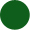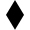# Complexity Explorer Santa Fe Institute

This course is no longer in session.## Nonlinear Dynamics: Mathematical and Computational Approaches (Spring 2020)

• Introduction to nonlinear dynamics
• Maps and difference equations
• Transients and attractors
• Parameters and bifurcations
• Field trip: Boulder Creek
• Maps I: Unit test
• Return maps
• Constructing the bifurcation diagram
• Exploring the bifurcation diagram
• Feigenbaum and universality
• Field trip: The standard map (with Jim Meiss)
• Maps II: Unit test
• What is a flow?
• State variables and state space
• Introduction to ordinary differential equations (ODEs)
• Nonlinearity and nonintegrability
• Field trip: ODEs and the human insulin system (with Sriram Sankaranarayanan)
• Flows I: Unit test
• Fixed points and stability
• Stable and unstable manifolds
• Attractors, strange and otherwise
• Field trip: Using stable and unstable manifolds to design spacecraft trajectories (with Jeff Parker)
• Flows II: Unit test
• ODEs, vector fields, and dynamical landscapes
• Introduction to ODE solvers
• Two simple ODE solvers: forward and backward Euler
• Solving the simple harmonic oscillator ODEs
• Field trip: Systems that can't be modeled with ODEs (with Jean Hertzberg)
• Flows III: Unit test
• ODE solvers, round II: Error and adaptation
• Production ODE solvers
• Numerical dynamics and due diligence
• Field trip: Solving partial differential equations (with Christine Hrenya)
• Flows IV: Unit test
• Dynamics and state-space deformation
• Lyapunov exponents
• Sections and projections
• Unstable periodic orbits
• Fractals and chaos
• Field trip: Diffusion-limited aggregation, fractals, and snowflakes (with Dave Feldman)
• Flows V: Unit test
• Time-series analysis and the observer problem
• Delay-coordinate embedding
• Topology, diffeomorphisms, and reconstruction of dynamics
• Estimating embedding parameters
• Caveats and extensions
• Field trip: Detecting extreme events (with Holger Kantz)
• Nonlinear time-series analysis I: Unit test
• Computing fractal dimensions
• Computing Lyapunov exponents
• Noise and filtering
• Field trip: Chaotic mixing and marine invertebrate reproduction (with John Crimaldi)
• Nonlinear time-series analysis II: Unit test
• Prediction
• Control of chaos
• Classical mechanics
• Music and dance (with a coda on the difference between chaos and complexity)
• Applications: Unit test

#### Quiz scores are NOT recorded.

• You may come back to quizzes and take them as many times as you like
• When you are finished, clicking the "Score" button at the bottom of the test will show you the correct responses.

#### Instructions

Homework problems in this course are broken into three types and marked by the following symbols:-- Mandatory problems that should be accessible to all students.-- Optional problems that are somewhat harder.  We strongly suggest that you work through these problems; completing them will give you greater insight into the topics at hand.  Please discuss them and their results on the forum.-- Challenging problems that are intended for experts. These are optional; not all students will be able to complete them. Again, feel free to discuss them on the forum.

In the first set of questions, you will use delay-coordinate embedding to reconstruct the dynamics from the time-series dataset called amplitude.dat (click on file name to download).